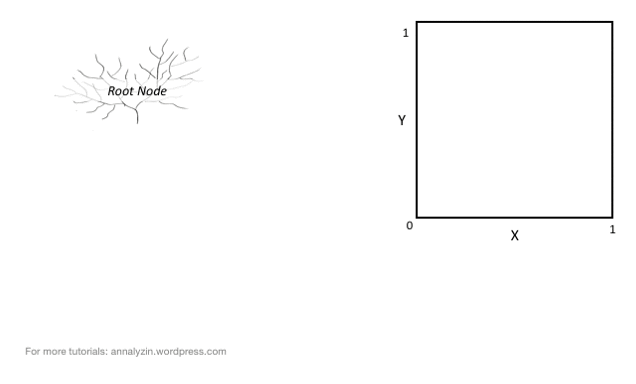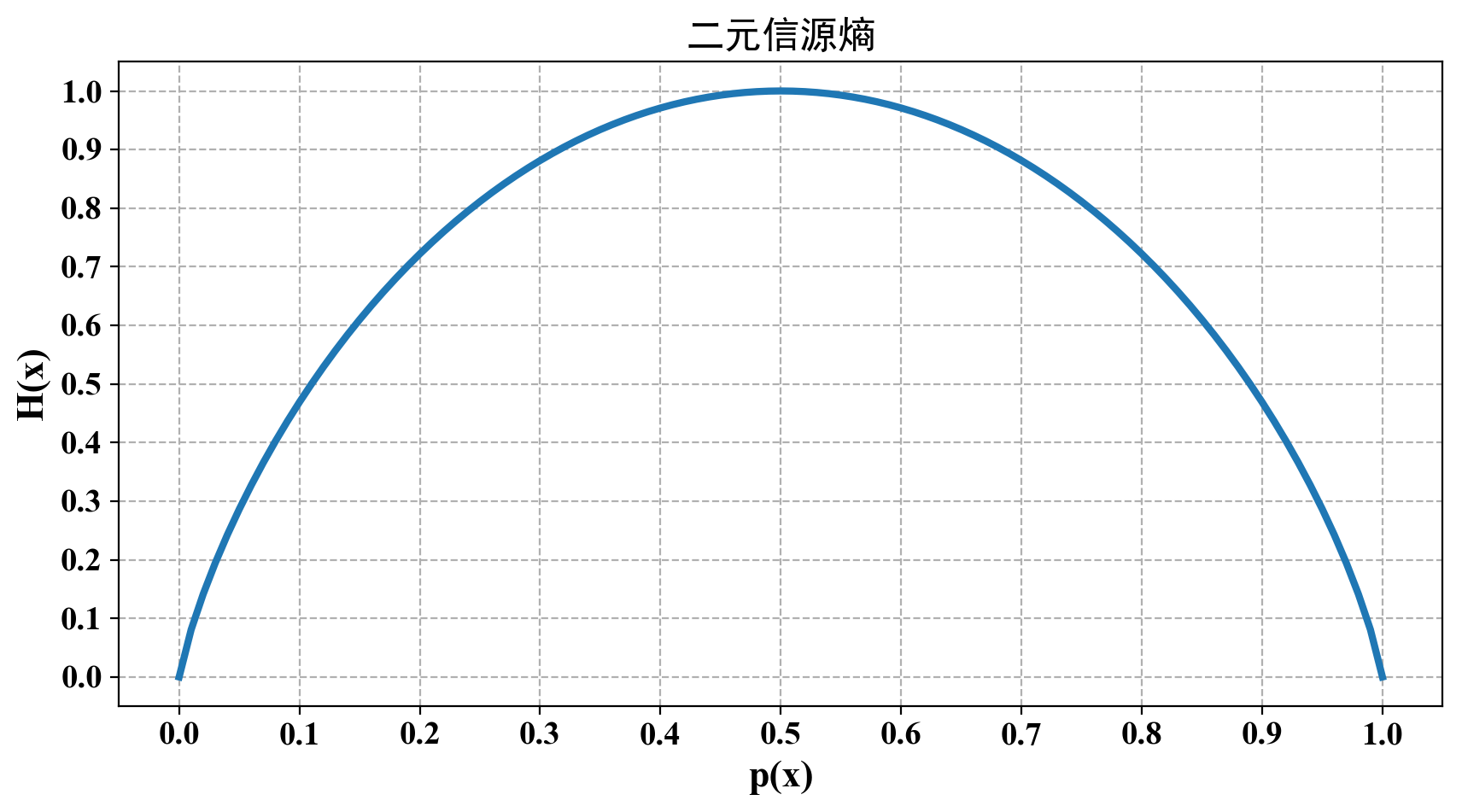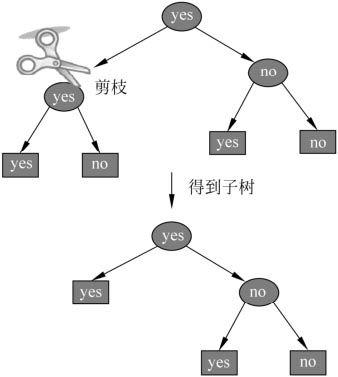## 基本原理

### 条件概率分布• 决策树的每一条路径对应于划分中的一个基本单元。
• 设某个单元$\mathbb S$内部有$N_S$个样本点，则它定义了一个条件概率分布$p(y\mid \mathbf{\vec x}), \mathbf{\vec x} \in \mathbb S$。
• 单元上的条件概率偏向哪个类，则该单元划归到该类的概率较大。即单元的类别为：$\arg\max_y p(y \mid \mathbf{\vec x}), \mathbf{\vec x} \in \mathbb S$
• 决策树所代表的条件概率分布由各个单元给定条件下类的条件概率分布组成。

## 特征选择

### 信息增益—般地，熵$H(Y)$与条件熵之差$H(Y\mid X)$称为互信息(Mutual Information)。决策树学习中的信息增益等价于训练数据集中类与特征的互信息。

​ 输入：训练数据集$D$和特征$A$；

​ 输出：特征$A$对训练数据集$D$的信息增益$g(D,A)$。

​ (1) 计算数据集$D$的经验熵$H(D)$：

​ (2) 计算特征$A$对数据集$D$的经验条件熵$H(D\mid A)$：

​ (3) 计算信息增益：

## 生成算法

### ID3算法

ID3算法的核心是在决策树各个结点上应用信息增益准则选择特征，递归地构建决策树。具体方法是：从根结点(Root Node)开始，对结点计算所有可能的特征的信息增益，选择信息增益最大的特征作为结点的特征，由该特征的不同取值建立子结点；再对子结点递归地调用以上方法，构建决策树；直到所有特征的信息增益均很小或没有特征可以选择为止。最后得到一个决策树。ID3相当于用极大似然法进行概率模型的选择。具体算法过程如下：

ID3的生成算法

​ 输入：训练数据集$D$，特征集$A$，阈值$\varepsilon$；

​ 输出：决策树$T$。

​ (1) 若$D$中所有实例属于同一类$C_{k}$，则$T$为单结点树，并将类作为该结点的类标记，返回$T$；

​ (2) 若$A= \varnothing$，则$T$为单结点树，并将$D$中实例数最大的类$C_{k}$作为该结点的类标记，返回$T$；

​ (3) 否则，计算$A$中各特征对$D$的信息增益，选择信息增益最大的特征$A_{g}$；

​ (4) 如果$A_{g}$的信息增益小于阈值$\varepsilon$，则置$T$为单结点树，并将$D$中实例数最大的类作为该结点的类标记，返回$T$；

​ (5) 否则，对$A_{g}$的每一可能值$a_{i}$，依$A_{g}=a_{i}$将$D$分割为若干非空子集$D_{i}$，将 $D_{i}$中实例数最大的类作为标记，构建子结点，由结点及其子结点构成树$T$，返回$T$。

​ (6) 对第i个子结点，以$D_{i}$为训练集，以为$A-\left \{ A_{g} \right \}$特征集，递归地调用步(1)〜步(5)，得到子树$T_{i}$，返回$T_{i}$。

ID3算法只有树的生成，所以该算法生成的树容易产生过拟合。

### C4.5算法

C4.5算法与ID3算法相似，C4.5算法对ID3算法进行了改进。C4.5在生成的过程中，用信息增益比来选择特征。

C4.5的生成算法

​ 输入：训练数据集$D$，特征集$A$，阈值$\varepsilon$；

​ 输出：决策树$T$。

​ (1) 若$D$中所有实例属于同一类$C_{k}$，则$T$为单结点树，并将类作为该结点的类标记，返回$T$；

​ (2) 若$A= \varnothing$，则$T$为单结点树，并将$D$中实例数最大的类$C_{k}$作为该结点的类标记，返回$T$；

​ (3) 否则，计算$A$中各特征对$D$的信息增益比，选择信息增益比最大的特征$A_{g}$；

​ (4) 如果$A_{g}$的信息增益比小于阈值$\varepsilon$，则置$T$为单结点树，并将$D$中实例数最大的类作为该结点的类标记，返回$T$；

​ (5) 否则，对$A_{g}$的每一可能值$a_{i}$，依$A_{g}=a_{i}$将$D$分割为若干非空子集$D_{i}$，将 $D_{i}$中实例数最大的类作为标记，构建子结点，由结点及其子结点构成树$T$，返回$T$。

​ (6) 对第i个子结点，以$D_{i}$为训练集，以为$A-\left \{ A_{g} \right \}$特征集，递归地调用步(1)〜步(5)，得到子树$T_{i}$，返回$T_{i}$。

## 剪枝算法​ 输入：生成算法产生的整个树$T$，参数$a$：

​ 输出：修剪后的子树$T$。

​ (1) 计算每个结点的经验熵；

​ (2) 递归地从树的叶结点向上回缩。设一组叶结点回缩到其父结点之前与之后的整体树分别为$T_{B}$和$T_{A}$，其对应的损失函数值分别是$C_{a}(T_{B})$与$C_{a}(T_{A})$，如果$C_{a}(T_{A}) \leqslant C_{a}(T_{B})$，则进行剪枝，即将父结点变为新的叶结点。

​ (3) 返回(2)，直至不能继续为止，得到损失函数最小的子树$T_{a}$。

## CART 树

CART是在给定输入随机变量X条件下输出随机变量Y的条件概率分布的学习方法。CART假设决策树是二叉树，内部结点特征的取值为“是”和“否”，左分支是取值为“是”的分支，右分支是取值为“否”的分支。这样的决策树等价于递归地二分每个特征，将输入空间即特征空间划分为有限个单元，并在这些单元上确定预测的概率分布，也就是在输入给定的条件下输出的条件概率分布。

CART算法由以下两步组成：

​ (1) 决策树生成：基于训练数据集生成决策树，生成的决策树要尽量大；

​ (2) 决策树剪枝：用验证数据集对己生成的树进行剪枝并选择最优子树，这时用损失函数最小作为剪枝的标准。

### CART 生成

1. 回归树的生成

1. 分类树的生成

CART生成算法

​ 输入：训练数据集$D$，停止计算的条件；

​ 输出：CART决策树。

​ 根据训练数据集，从根结点开始，递归地对每个结点进行以下操作，构建二叉决策树：

​ (1) 设结点的训练数据集为$D$，计算现有特征对该数据集的基尼指数。此时，对每一个特征对其可能取的每个值$a$，根据样本点对$A=a$的测试为“是”或 “否”将$D$分割成$D_{1}$和$D_{2}$两部分，计算$A=a$时的基尼指数。

​ (2) 在所有可能的特征$A$以及它们所有可能的切分点$a$中，选择基尼指数最小的特征及其对应的切分点作为最优特征与最优切分点。依据最优特征与最优切分点，从现结点生成两个子结点，将训练数据集依特征分配到两个子结点中去。

​ (3) 对两个子结点递归地调用上述操作，直至满足停止条件为止。

​ (4) 生成CART决策树。

### CART 剪枝

CART剪枝算法从“完全生长”的决策树的底端剪去一些子树，使决策树变小（模型变简单），从而能够对未知数据有更准确的预测，提高模型泛化能力。CART剪枝算法由两步组成：首先从生成算法产生的决策树$T_{0}$底端开始不断剪枝，直到$T_{0}$的根结点，形成一个子树序列$\left \{ T_{0},T_{1}, \cdots ,T_{n} \right \}$；然后通过交叉验证法在独立的验证数据集上对子树序列进行测试，从中选择最优子树。

t为根结点的子树$T_{t}$的损失函数是：

## 参考文献

-------------本文结束感谢您的阅读-------------

【如果你觉得本文还不错，对你的学习带来了些许帮助，赞赏是对作者最好的鼓励】

0%